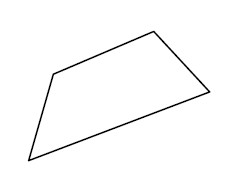### Home > MC2 > Chapter 5 > Lesson 5.3.3 > Problem5-111

5-111.Alexa is looking at the shape below. She needs to make several different figures that are similar to it. .

1. If she uses the scale factor $\frac { 8 } { 5 }$, will the new shape that she creates be larger or smaller than the original? Justify your answer.

When multiplying by a number larger than $1$, will the new number be larger or smaller than it originally was?
Consider multipliers in terms of scale factors.

2. List two different scale factors Alexa could use to make a smaller shape.

Any number less than $1$, but greater than $0$.

3. List two different scale factors Alexa could use to make a larger shape.

Any number greater than $1$.

Explore using the eTool below.
Click the link at right for the full eTool version: MC2 5-111 HW eTool